## -Electromagnetism-

When we studied magnetism it was mentioned that a magnetic field is produced when a charge moves. Since an electric current is a flow of charged particles it follows that a magnetic field surrounds a current carrying wire.

### Magnetic Field around a current carrying conductor

#### A Long Straight Conductor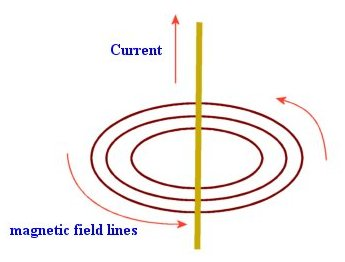Isometric View of the Magnetic lines of force around a long straight conductor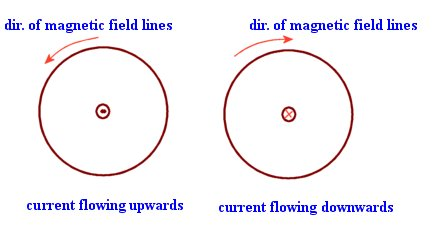Top View of the Magnetic lines of force around a long straight conductor

The lines of magnetic flux around a long straight current carrying wire are concentric circles with the conductor passing through the circles center. The direction of the field is taken as the direction of force on a north pole if placed in the field. The diagrams show that if the current is flowing towards the observer then the direction of the field lines is counterclockwise.  If the current is flowing away from the observer then the field lines are clockwise.

Note that the lines of force lie in a plane that is perpendicular to the direction of the current.

The Corkscrew rule:

If we were to drive a screw in the direction of the current then the direction of rotation of the screw is the direction of the lines of force.

Alternatively you may choose to remember that if you grasp the conductor, with your right hand, with your thumb pointing in the direction of the current (and outwards from your palm - in a comfortable manner) then your other fingers gives the orientation (clockwise or anticlockwise) of the lines of force. This is called the Right Hand Grip Rule.

##### An Aside - 3D Spatial coordinates (Optional)

We can relate these two rule to the right hand set of base vectors used in the 3D Cartesian system.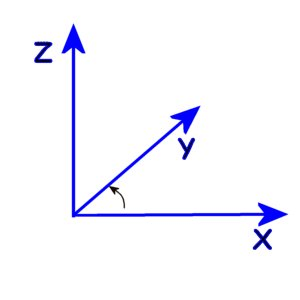RH Cartesian Base Vectors (3D space)

The base vectors for the horizontal plane are the x and y axes which are perpendicular to each other.  The z axis  is perpendicular to the horizontal plane as well as both the x and y axes. (It is a vertical line directed upwards). The corkscrew rule and the right hand grip rule can be related to the base vectors of Cartesian system. If the lines of flux rotate from the positive x-axis to the positive y-axis then the current (that established the magnet field) flows in the direction of the positive z-axis.

Not convinced? Superimpose the Isometric View of the Magnetic lines of force around a long straight conductor figure with the diagram labeled RH Cartesian Base Vectors (3D space).

#### A Single coil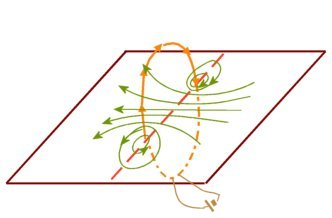Magnetic field of a single narrow coil

The direction of current and resulting magnetic field is clearly depicted in the diagram. Either the right hand grip rule or the corkscrew rule can be used to derive the direction of the field based on the direction of the current in the coil.

At the end of the coil nearer to the observer, the current is flowing upwards giving an anticlockwise field. At the end furthest from the observer, the current is flowing downwards giving a clockwise field. These fields interact constructively giving the overall field shown. Observe how the flux lines are straighter nearer the centre of the coil.

#### A Solenoid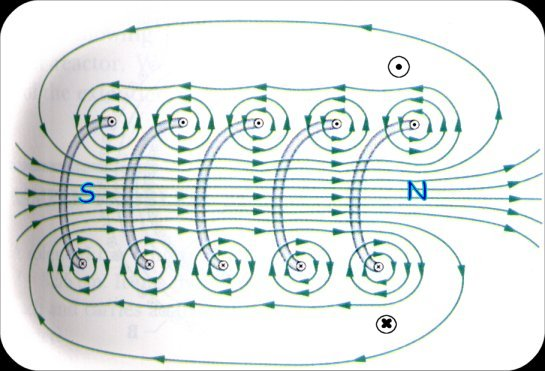Magnetic field of a solenoid (Expanded)

The solenoid above is expanded to show how the total field results from the interaction of the fields of individual coils. (Observe how the field is similar to that of a bar magnet.)

The right hand grip rule can be used to determine the direction of the field. Hold the coil with the fingers curled around it in the direction of the current. The extended thumb points in the direction on the field. (For the coil above the fingertips are nearer the observer and pointing downwards while the thumb is pointing towards the right, which is the north pole.

##### Meaning of Curl ? (Optional)

In Mathematics there is a vector product called Curl.

Curl a = del × a

a × b = c

Suppose that a is in the positive x direction and b is in the positive y direction then "a cross b", c, is in direction of the positive z- axis. Using the RH grip rule, curling your fingers from a (x) to b (y), the thumb point towards c (z)

Note Well: No text that I have come across  mentions the simple truth of right hand and left hand coils. A right hand coil is a helix that moves away from the observer as you move clockwise along the coil. A left hand coil moves is a helix that moves towards the observer as you move clockwise along the coil. Thus if a RH coil is used as a solenoid then the North pole is is end that is connected to the Negative terminal of the battery. Conversely, for a LH coil the north pole is the end that is connected to the positive terminal of the battery.

Verify this by using the Right Hand grip rule.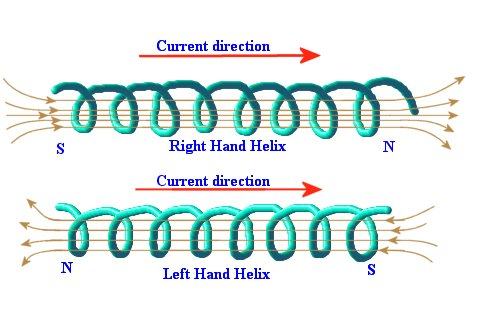Magnetic fields for RH and LH coils

### Problems:

1. How do we represent a current flowing towards an observer? (Hint: Circular wire cross-section)
2. How do we represent current flowing away from the observer?
3. Sketch the field around a single long conductor showing the current and field directions. State the rule that you used to determine the direction of the field.
4. A solenoid has the form of a RH helix. What pole is formed at the end of the coil connected to the positive terminal of the battery?
5. Sketch the field around the solenoid of the previous question.